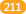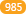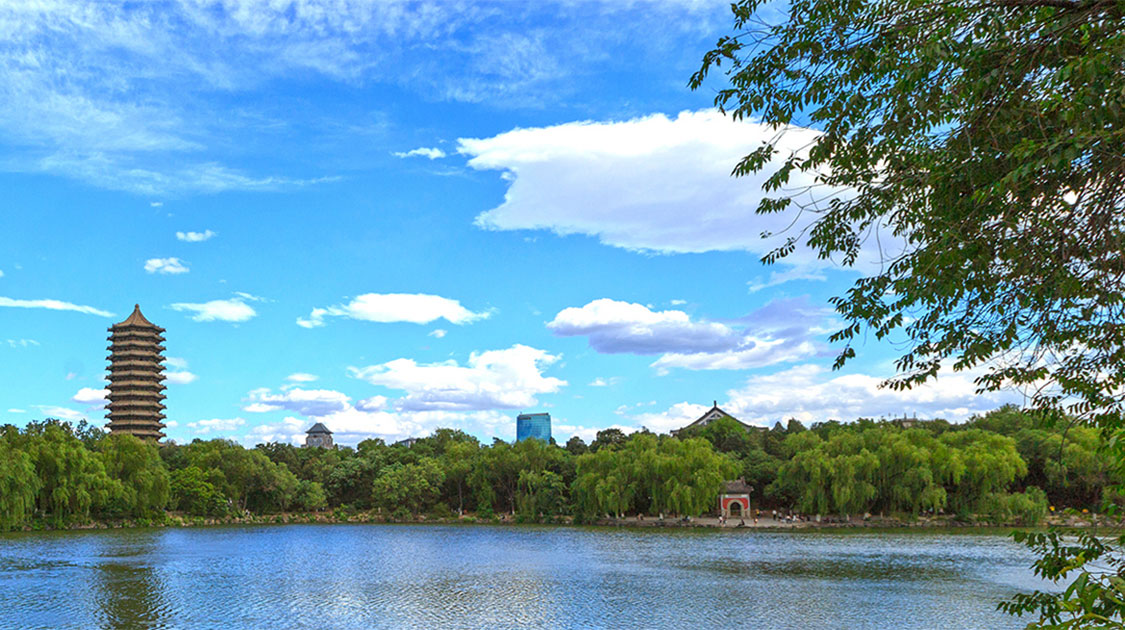## 北京大学全景校园

### Peking University•1898

•公办

•综合

•教育部

•本科

•北京-海淀区

•333个

•307个

### 院校分数线

多方向招生### 特色专业### 院系/专业

• 化学（本）
• 材料化学（本）
• 应用化学（本）
• 化学生物学（本）

• 环境科学（本）
• 自然地理与资源环境（本）
• 生态学（本）
• 人文地理与城乡规划（本）
• 城乡规划（本）

• 应用统计学（本）
• 数据科学与大数据技术（本）
• 统计学（本）
• 数学与应用数学（本）
• 信息与计算科学（本）

• 地质学（本）
• 地球物理学（本）
• 空间科学与技术（本）
• 地球化学（本）
• 地理信息科学（本）

• 物理学（本）
• 大气与海洋科学（本）
• 天文学（本）
• 核物理（本）

• 应用心理学（本）
• 心理学(基础心理学)（本）

• 生物技术（本）
• 生物科学（本）

• 计算机科学与技术（本）
• 电子信息科学与技术（本）
• 微电子科学与工程（本）
• 智能科学与技术（本）

• 环境科学（本）
• 环境工程（本）

• 国际政治（本）
• 国际政治(国际组织与国际公共政策专业方向)（本）
• 国际政治(国际政治经济学专业方向)（本）
• 外交学（本）

• 汉语言（本）
• 汉语言文学（本）
• 中国语言文学类(中国文学)（本）
• 古典文献学（本）
• 应用语言学（本）

• 航空航天工程（本）
• 理论与应用力学（本）
• 工程力学(工程结构分析方向)（本）
• 机器人工程（本）
• 材料科学与工程（本）
• 工科试验班类(能源与资源工程)（本）
• 生物医学工程（本）

• 文物与博物馆学（本）
• 考古学(文物建筑方向)（本）
• 考古学（本）
• 文物保护技术（本）

• 保险学（本）
• 国际经济与贸易（本）
• 金融学（本）
• 财政学（本）
• 资源与环境经济学（本）
• 经济学（本）

• 英语（本）
• 泰语（本）
• 法语（本）
• 缅甸语（本）
• 西班牙语（本）
• 乌尔都语（本）
• 阿拉伯语（本）
• 波斯语（本）
• 俄语（本）
• 印地语（本）
• 菲律宾语（本）
• 朝鲜语（本）
• 印度尼西亚语（本）
• 越南语（本）
• 蒙古语（本）
• 日语（本）
• 梵语巴利语（本）
• 德语（本）
• 希伯来语（本）
• 葡萄牙语（本）

• 宗教学（本）
• 哲学(科哲与逻辑学方向)（本）
• 哲学（本）

• 历史学（本）
• 世界史（本）

• 艺术史论（本）
• 戏剧影视文学（本）
• 文化产业管理（本）

• 社会学（本）
• 人类学（本）
• 社会工作（本）

• 图书馆学（本）
• 信息管理与信息系统（本）

• 城市管理（本）
• 行政管理（本）
• 政治学与行政学（本）

• 广播电视学（本）
• 广告学（本）
• 新闻学（本）
• 编辑出版学（本）

• 马克思主义理论（本）

• 市场营销（本）
• 金融学（本）
• 会计学（本）
• 工商管理（本）

• 法学（本）

• （本）

### 大学排名 2020 2019 2018

QS

USNews### 知名校友

•李彦宏

•俞洪敏

•撒贝宁

•马云